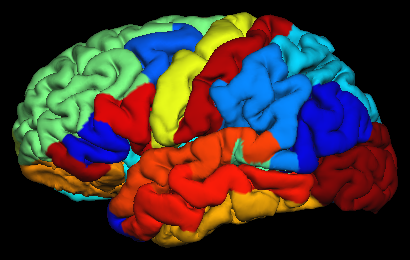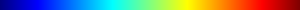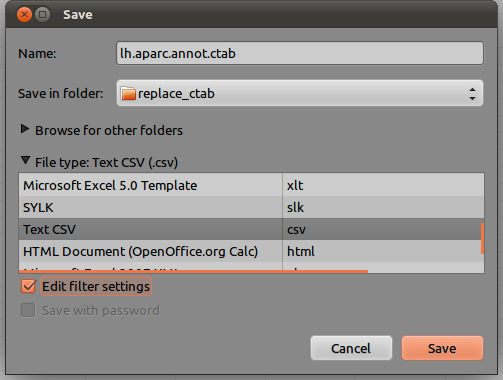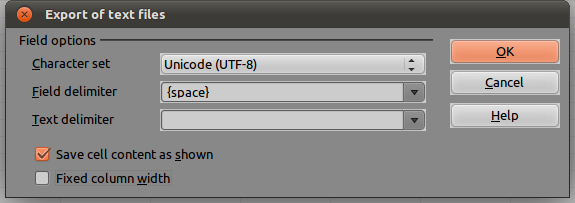# Converting OASIS brains to NIFTI

The OASIS dataset consists of a number of T1-weighted mri brain scans, which has been kindly offered online at http://www.oasis-brains.org. The dataset can be downloaded for free after accepting an academic agreement available at the website. The data was released in analyze 7.5 file format. This format, despite having been used by the neuroimaging community for many years, suffers from not including orientation information. The nifti format addresses this concern, but when converting, some care has to be taken to ensure that there are no left-right flips. Fortunately, for this dataset, during acquisition a capsule of vitamin E was placed next to the head of each subject, on the left side, which is very helpful to identify the correct side (Marcus et al., 2007).

There are still problems, though. The nifti format has not been fully implemented in all common software packages and worse, some packages interpret differently the information contained in the header. Images that look fine in fsl‘s FSLview or FreeSurfer‘s Freeview may look stretched or shrunken in spm for instance. And images that look fine in both, may still be oriented incorrectly in Mango. Although a bit cumbersome, the procedure below ensures that the oasis images can be converted from analyze to nifti in a way that it can correctly read and shown by Mango, fsl, FreeSurfer and spm. The procedure uses exclusively fsl tools:

1. Convert to nifti:
fslchfiletype NIFTI_GZ OAS1_0001_MR1_mpr-1_anon.hdr
2. Make sure there is no undesired orientation information:
fslorient -deleteorient OAS1_0001_MR1_mpr-1_anon.nii.gz
3. Set the sform_code as 2, which is for “aligned anatomy”. Although this is still in native, not aligned space, it ensures that software will read them appropriately:
fslorient -setsformcode 2 OAS1_0001_MR1_mpr-1_anon.nii.gz
4. Set the sform as the following matrix:
fslorient -setsform  0 0 -1.25 0  1 0 0 0  0 1 0 0  0 0 0 1  OAS1_0001_MR1_mpr-1_anon.nii.gz
5. Swap the order of the data. Again, this isn’t really necessary, except to ensure that different applications will all read correctly:
fslswapdim OAS1_0001_MR1_mpr-1_anon.nii.gz RL PA IS OAS1_0001_MR1_mpr-1_anon.nii.gz
6. fsl tries to preserve orientation and, when the voxels are reordered, it modifies the header accordingly, resulting in no net transformation when seen with fsl tools. To resolve this, it’s necessary to change the header again, now the qform:
fslorient -setqform -1.25 0 0 0  0 1 0 0  0 0 1 0  0 0 0 1  OAS1_0001_MR1_mpr-1_anon.nii.gz

These steps can be placed inside a simple loop within the shell for either Linux and Mac, like below (click here to download):

#!/bin/bash

# Directory where the OASIS data is located
ROOTDIR=/Volumes/HD2/oasis-dataset-416subj/original

# Directory to save results
NIFTIDIR=${ROOTDIR}/../nifti # Go to the directory with the data cd${ROOTDIR}

# For each subject
for s in * ; do

# Some feedback in the screen
echo ${s} # Create directory to save the results, if not existing mkdir -p${NIFTIDIR}/${s} # Directory of the original, raw data cd${ROOTDIR}/${s}/RAW # For each acquisition for a in *.hdr ; do # Do each of the 6 steps described in the blog${FSLDIR}/bin/fslchfiletype NIFTI_GZ ${a}${NIFTIDIR}/${s}/${a%.hdr}
${FSLDIR}/bin/fslorient -deleteorient${NIFTIDIR}/${s}/${a%.hdr}
${FSLDIR}/bin/fslorient -setsformcode 2${NIFTIDIR}/${s}/${a%.hdr}
${FSLDIR}/bin/fslorient -setsform 0 0 -1.25 0 1 0 0 0 0 1 0 0 0 0 0 1${NIFTIDIR}/${s}/${a%.hdr}
${FSLDIR}/bin/fslswapdim${NIFTIDIR}/${s}/${a%.hdr} RL PA IS ${NIFTIDIR}/${s}/${a%.hdr}${FSLDIR}/bin/fslorient -setqform -1.25 0 0 0  0 1 0 0  0 0 1 0  0 0 0 1  ${NIFTIDIR}/${s}/${a%.hdr} done done echo "Done!"  The reference for the oasis dataset is: # FreeSurfer brains in arbitrary colours Suppose you run a statistical test for each of the regions of the parcellated brain produced by FreeSurfer. It can be either surface area comparison between groups, or maybe differences in thickness. For each region, you may obtain a statistical score and likely a p-value as well. How to display these results in a color-coded brain model, still inside FreeSurfer?The process is consists of three steps: 1. Create of a table with your results, in a format that FreeSurfer can read. 2. Embed the table in an annotation file. 3. Display in tksurfer. ## 1. Create a table The table must contain not the actual scalars, but instead rgb triplets. This can be done using a simple Octave or matlab script. Since often these results are tabulated as spreadsheets, an alternative, straightforward way is using software like LibreOffice, which gives instantaneous graphical feedback of how the resulting table looks like. An example of such a spreadsheet is this: colormap.ods. The data go into the sheets named lh.data and rh.data. For structures that are not supposed to display any color, put an off-range value below the minimum value of the other regions (e.g. -1 if the scalars are all positive). Do not add or remove structures. The sheet named colormap contains the key between the scalar values and the actual rgb colors. In this sheet, the first column (column A) is simply a rank number, used in the calculations; the second column (col. B) contains the range of values between 0 and max, regularly spaced. The remaining three columns (C, D and E) contains the rgb triplets to be assigned to each value in column B. For each scalar to be shown, the closest number has its corresponding rgb used. The sheets named lh.aparc.annot.ctab and rh.aparc.annot.ctab contain the resulting tables that will be used for actual display purposes. Each contain an index number, starting from 0, the structure name as used in FreeSurfer, the rgb colors, and a fourth value that is called “flag” and is usually zero. In most cases, only the numerical values in lh.data and rh.data have to be changed. In some occasions, it may be necessary to change the range to be shown (i.e., column B on the colormap sheet), or the colormap itself (columns C, D and E). Different colormaps can be generated, for instance, using Octave. In the file used here, the colormap is the “spectrum”, also known as “jet”, shown below:Finally, FreeSurfer cannot read binary spreadsheets like this. The file has to be converted to simple values separated by space. In LibreOffice or OpenOffice, there is a very simple way of doing this. Go to File -> Save As… In the File type, choose Text CSV, and make sure to mark the option Edit Filter Settings:On the next window, as Field Delimiter, choose {space}. As Text delimiter, leave blank, as shown below:The resulting file, saved to the disk, should look like this (click here for the full file):  0 bankssts 92 255 163 0 1 caudalanteriorcingulate 143 255 112 0 2 caudalmiddlefrontal 0 92 255 0 [...] 34 transversetemporal 255 133 0 0 35 unknown 160 160 160 0  ## 2. Embed the table into annotation file Once a table has been produced, it can be embedded into the annotation file that defines the the vertices that belong to each region. The annotation files produced by FreeSurfer already contain a default color table, which can be replaced. This can be accomplished by the Octave function replace_ctab.m. This function needs Octave 3.4.0+ or matlab. Type help replace_ctab at the prompt to get usage information. Here is an example: replace_ctab('${SUBJECTS_DIR}/bert/label/lh.aparc.annot',...
'${SUBJECTS_DIR}/bert/label/lh.aparc.annot.ctab.csv',... '${SUBJECTS_DIR}/bert/label/lh.aparc.annot.new');


In this example, the original annotation is stored in the file ${SUBJECTS_DIR}/bert/label/lh.aparc.annot, the new color table, as saved in LibreOffice, is in the file ${SUBJECTS_DIR}/bert/label/lh.aparc.annot.ctab. The new annotation file, with the new colortable, will be in the file ${SUBJECTS_DIR}/bert/label/lh.aparc.annot.new. Notice that for the function replace_ctab to work, the directory ${FREESURFER_HOME}/matlab must be in the Octave or matlab path.

## 3. Display with tksurfer

Once the new annotation file has been produced, display the result is straightforward:

tksurfer bert lh pial -annotation lh.aparc.annot.new


The result should be like the brain shown at the beginning of this article.

Update (01.Aug.2013): In more recent FS versions, the “lh” in front of the annotation file can be omitted, as it’s already specified in the same command line (thanks to Krishna Pancholi, Olin Neuropsychiatric Research Center, for the tip.). This affects only the last command line, which becomes then:

tksurfer bert lh pial -annotation aparc.annot.new


# Normality tests I: Brief overview

Before the fast computers era, it was difficult to test how valid certain statistical methods were. Even in the 1970’s and 1980’s, when computers were already available at Universities and even at home, certain matrix operations were limited by hardware constraints. We are now at a much more comfortable position to analyse how these methods perform. One area where (nowadays) simple computer runs can be illuminating is with respect to normality tests. Tests to evaluate normality or a specific distribution, frequentist approaches can be broadly divided into two categories:

1. Tests based on comparison (“best fit”) with a given distribution, often specified in terms of its cumulative distribution funtion (cdf). Examples are the Kolmogorov-Smirnov test, the Lilliefors test, the Anderson-Darling test, the Cramér-von Mises criterion, as well as the Shapiro-Wilk and Shapiro-Francia tests.
2. Tests based on descriptive statistics of the sample. Examples are the skewness test, the kurtosis test, the D’Agostino-Pearson omnibus test, the Jarque-Bera test.

In this article I’ll briefly review six well-known normality tests: (1) the test based on skewness, (2) the test based on kurtosis, (3) the D’Agostino-Pearson omnibus test, (4) the Shapiro-Wilk test, (5) the Shapiro-Francia test, and (6) the Jarque-Bera test. In a subsequent article, I’ll analyse the analytical p-value approximations for these tests, and in a third article, I’ll provide Monte Carlo critical values for each of them.

## Skewness test

The skewness of the sample data can be converted to an useful score to evaluate normality with the following transformation (D’Agostino, 1970):

1. Compute the sample skewness$\sqrt{b_1} = m_3/m_2^{3/2}$, where$m_k=\sum_i (X_i-\bar X)^k/n$ is the k-th moment,$\bar X=\sum_i X_i/n$ is the sample mean, and$n$ is the sample size.
2. Compute:$Y = \sqrt{b_1}\left(\frac{(n+1)(n+3)}{6(n+2)}\right)^{1/2}$$\beta_2(\sqrt{b_1}) = \frac{3(n^2+27n-70)(n+1)(n+3)}{(n-2)(n+5)(n+7)(n+9)}$$W^2 = -1+(2(\beta_2(\sqrt{b_1})-1))^{1/2}$$\delta = 1/\sqrt{\ln W}$$\alpha = (2/(W^2-1))^{1/2}$
3. Compute:$Z(\sqrt{b_1}) = \delta \ln(Y/\alpha + ((Y/\alpha)^2+1)^{1/2})$

The$Z(\sqrt{b_1})$ is the test statistic and it is approximately normally distributed under the null hypothesis that the population data follows a normal distribution.

## Kurtosis test

Like the test based on skewness, the test based on kurtosis needs that the sample kurtosis is calculated, then transformed, and finally converted to a p-value. The steps are (Anscombe & Glynn, 1983):

1. Compute the sample kurtosis$b_2=m_4/m_2^2$, where$m_k=\sum_i (X_i-\bar X)^k/n$ is the k-th moment,$\bar X=\sum_i X_i/n$ is the sample mean, and$n$ is the sample size.
2. Compute:$\mathsf{E}\{b_2\} = 3(n-1)/(n+1)$$\mathsf{var}\{b_2\} = \frac{24n(n-2)(n-3)}{(n+1)^2(n+3)(n+5)}$$x = (b_2-\mathsf{E}\{b_2\})/\sqrt{\mathsf{var}\{b_2\}}$$\sqrt{\beta_1(b_2)}=\frac{6(n^2-5n+2)}{(n+7)(n+9)}\sqrt{\frac{6(n+3)(n+5)}{n(n-2)(n-3)}}$$A = 6+\frac{8}{\sqrt{\beta_1(b_2)}}\left(\frac{2}{\sqrt{\beta_1(b_2)}}+\sqrt{1+\frac{4}{\beta_1(b_2)}}\right)$, where$\mathsf{E}\{\cdot\}$ and$\mathsf{var}\{\cdot\}$ represent respectively the expected value and the variance.
3. Compute:$Z(b_2) = \left(\left(1-\frac{2}{9A}\right)-\left(\frac{1-2/A}{1+x\sqrt{2/(A-4)}}\right)^{1/3}\right)/\sqrt{\frac{2}{9A}}$

The$Z(b_2)$ is the test statistic and is considered approximately normally distributed under the null hypothesis that the population data follows a normal distribution.

## D’Agostino-Pearson omnibus test

The skewness and kurtosis tests can be combined to produce a single, global, “omnibus” statistic. This global test has been proposed by D’Agostino and Pearson (1973) and its statistic is simply$K^2=(Z(\sqrt{b_1}))^2 + (Z(b_2))^2$. In other words, simply square the statistics from the skewness and kurtosis tests and sum them together. The distribution of the$K^2$ statistic is approximately a$\chi^2$ distribution with two degrees of freedom under the null hypothesis that the sample was drawn from a population with normally distributed values. The skewness, kurtosis and the D’Agostino-Pearson tests have been collectively reviewed and discussed in D’Agostino et al. (1990).

A matlab/Octave implementation of the D’Agostino-Pearson test is available here: daptest.m.

## Jarque-Bera test

In a certain regard, this test, introduced by Jarque & Bera (1980) and later discussed in more detail in Jarque & Bera (1987), could also be called an “omnibus” test, that combines skewness and kurtosis into a single statistic. The test statistic is given by$\text{\textsc{lm}}= n\left(\frac{(\sqrt{b_1})^2}{6} + \frac{(b_2-3)^2}{24}\right)$. The$\text{\textsc{lm}}$ is asymptotically distributed as a$\chi^2$ distribution with two degrees of freedom under the null hypothesis that the sample was drawn from a population with normally distributed values.

A matlab/Octave implementation of the Jarque-Bera test is available here: jbtest.m.

## Shapiro-Wilk test

The Shapiro & Wilk (1965) test depends on the covariance matrix between the order statistics of the observations. Values for this covariance matrix have been laboriously tabulated for small samples (Sahan & Greenberg,1956) and algorithms have been developed (Davis & Stephens, 1978; Royston, 1982). In an effort to produce equivalents, yet computationally affordable results, it has been suggested to use approximations. The description here follow the approximation suggested by Royston (1992).

1. Sort the data in ascending order.
2. Compute the sample mean$\bar X=\sum_i X_i/n$, where$n$ is the sample size.
3. Compute the Blom scores (Blom, 1958) as$\tilde m_i = \Phi^{-1}\{(i-3/8)/(n+1/4)\}$, where$i$ is the rank order and$\Phi^{-1}\{\cdot\}$ represents the inverse normal cdf.
4. Compute a set of weights$c_i = \tilde m_i \sqrt{(\mathbf{\tilde m}'\mathbf{\tilde m})}$. These weights are the same used in the Shapiro-Francia test (see below).
5. Compute$\tilde a_n= - 2.706056u^5 + 4.434685u^4 - 2.071190u^3 - 0.147981u^2 + 0.221157u + c_n$, where$u=n^{-1/2}$. If$n>5$, compute also$\tilde a_{n-1}= -3.582633u^4 + 5.682633u^4 -1.752461u^3 -0.293762u^2 + 0.042981u + c_{n-1}$.
6. Compute$\phi = (\mathbf{\tilde m}'\mathbf{\tilde m} - 2\tilde m_n^2)/(1-2\tilde a_n^2)$ if$n \leq 5$ or$\phi = (\mathbf{\tilde m}'\mathbf{\tilde m} - 2\tilde m_n^2 - 2\tilde m_{n-1}^2)/(1-2\tilde a_n^2-2\tilde a_{n-1}^2)$ otherwise.
7. Compute the remaining$\tilde a_i = \phi^{-1/2} \tilde m_i$ for$i=2, \ldots ,n-1$ if$n \leq 5$ or$i=3,\ldots ,n-2$ if$n>5$.
8. Compute the test statistic$W=\left(\sum_i \tilde a_i X_i \right)^2 / \sum_i (X_i-\bar X)^2$.

Once the test statistic$W$ has been produced, it can be approximated through a function$g(W)$ to a normal distribution with mean$\mu$ and standard deviation$\sigma$ (Royston, 1993). For sample sizes between 4 and 11 (inclusive),$g(W)=-\ln(\gamma-\ln(1-W))$, where$\gamma=0.459n-2.273$. For sample sizes equal to or larger than 12,$g(W)=\ln(1-W)$.

For$4 \leq n \leq 11$, the parameters of the statistic transformed (normalized) by$g(W)$ are given by$\mu=-0.0006714n^3 + 0.025054n^2 -0.39978n + 0.5440$ and$\sigma=\mathsf{exp}\{-0.0020322n^3+ 0.062767n^2 -0.77857n + 1.3822\}$. For$n \geq 12$,$\mu = 0.0038915u^3 -0.083751u^2 -0.31082u -1.5851$ and$\sigma = \mathsf{exp}\{0.0030302u^2 -0.082676u -0.4803\}$, where$u = \ln(n)$.

A$Z$ statistic can then be produced trivially by$(g(W)-\mu)/\sigma$, and the p-values can be obtained from the normal cdf.

A matlab/Octave implementation of the Shapiro-Wilk test is available here: swtest.m.

## Shapiro-Francia test

The test was proposed by Shapiro & Francia (1972) as a simplification of the Shapiro-Wilk test. The simplification consisted in replacing the covariance matrix of the order statistics by the identity matrix. The test is generally considered asymptotically equivalent to the Shapiro-Wilk test for large and independent samples. To apply the test, the steps are (Royston, 1993):

1. Sort the data in ascending order.
2. Compute the sample mean$\bar X=\sum_i X_i/n$, where$n$ is the sample size.
3. Compute the Blom scores (Blom, 1958) as$\tilde m_i = \Phi^{-1}\{(i-3/8)/(n+1/4)\}$, where$i$ is the rank order and$\Phi^{-1}\{\cdot\}$ represents the inverse normal cumulative distribution function (cdf).
4. Compute a set of weights$c_i = \tilde m_i \sqrt{(\mathbf{\tilde m}'\mathbf{\tilde m})}$.
5. Compute the test statistic$W'=\left(\sum_i c_i X_i \right)^2 / \sum_i (X_i-\bar X)^2$.

Similarly as for the Shapiro-Wilk test, once the test statistic$W'$ has been produced, it can be approximated through a function$g(W')=\ln(1-W')$ to a normal distribution with mean$\mu$ and standard deviation$\sigma$ (Royston, 1993). The parameters of the statistic transformed (normalized) by$g(W')$ are given by$\mu=1.0521u - 1.2725$, where$u = \ln(\ln(n))-\ln(n)$ and$\sigma=-0.26758v+1.030$, where$v = \ln(\ln(n))+2/\ln(n)$. These approximations are valid for samples between 5 and 5000 at least.

A$Z$ statistic can then be produced trivially by$(g(W')-\mu)/\sigma$, and the p-values can be obtained from the normal cdf.

A matlab/Octave implementation of the Shapiro-Francia test is available here: sftest.m.